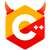# 1820. Hanoi Tower II### [EOJ 1820] Hanoi Tower II

$$f(i) = 2g(i-1) + 1$$

1. 把 $i-1$ 个盘子移到 $C$ 上，只有这样才能移动底盘。
2. 把底盘移到 $B$ 上。
3. 把 $i-1$ 个盘子移到 $A$ 上，只有这样才能再次移动底盘。
4. 把底盘移到 $C$ 上。
5. 把 $i-1$ 个盘子移到 $C$ 上。

$$g(i) = g(i-1) + 1+f(i-1)+1+g(i-1) = 2g(i-1)+f(i-1)+2$$

$$g(i) = f(i) + f(i-1) + 1$$